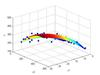linear regression

• ## 线性回归

千次阅读 2018-07-11 01:08:40
这篇博客为大家介绍线性回归模型，也是本人写的第一篇回归模型的博客。之前介绍的模型预测结果都是离散值，也就是机器学习当中的分类问题，那么机器学习中还有一大类预测问题，其预测结果为连续值，我们称之为回归...
这篇博客为大家简单介绍线性回归模型，也是写的第一篇回归模型的博客。之前介绍的模型预测结果都是离散值，也就是机器学习当中的分类问题，那么机器学习中还有一大类预测问题，其预测结果为连续值，我们称之为回归问题。线性回归属于机器学习中最基础的回归模型，因此，首先介绍线性回归模型。虽然线性回归模型是最基础的模型，但是其重要性也是不容小觑的。在很多实际项目应用当中，其效果还是不错的。首先我们用一个案例来引出线性回归：假设我们要预测某个房子的价格，那么我们如何去预测得知房屋的价格呢？通常情况下我们会综合考虑各个因素之后再给出这个房子的估价。这些因素有可能是房屋面积，卧室数目，房屋所处楼层，是否为学区房等等。当我们掌握了这些信息之后我们便能够基本预测得知这个房子的大概的价格。然而这只是凭借我们的经验来进行预测得知的价格，实际上我们并不知道这些因素同房价之间的真正的关系。线性回归要解决的问题就是找到这些因素同房价之间的真实关系，在已知其关系的情况下，我们就能根据已知的这些因素去预测得到房屋的价格。上文中所说的因素也就是机器学习中的样本的特征。如下表所示：房屋面积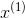卧室数目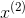所处楼层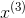是否学区房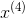房屋价格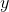123321100000090260600000表中每一行为一个房屋的特征信息以及价格信息，我们称之为一个样本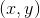，其中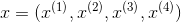代表房屋的各个特征。假设房屋价格与各个特征之间的关系为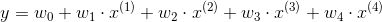，设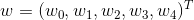，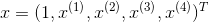，那么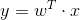，这便是线性回归的基本模型，注意，线性回归并不是指关于特征是线性的，而是说关于权重向量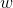是线性的，我们的任务就是要寻找最优的权重向量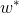，从而确定特征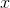和标记之间的关系。当确定后，则线性回归模型也就确定，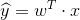，其中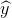为样本的预测值。接下来我们就来探索如何求得最好的：假设存在样本集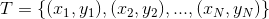，其中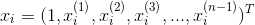为第i个样本的特征向量，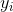为样本的真实标记。现在我们已知存在一组初始的权重向量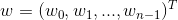，构造出了一个初始线性模型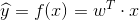，但是这个模型的预测效果并不是很好，因此我们需要对其进行优化。在当前模型下，给定样本特征向量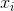，我们可以得到其预测值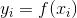。因此，我们也可以得到其预测误差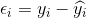。在这里我们假设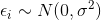，则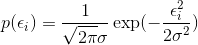为的概率密度函数，将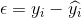带入得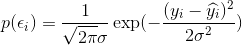。由于上式与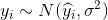时的概率密度一致，因此我们可以重新解释上式为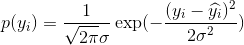。由此便可以的出似然函数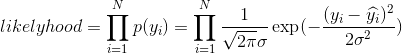，可以看出似然函数中的变量只有，我们只需要找出使得似然函数最大的权重向量即可，即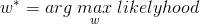。由于似然函数中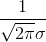为常数，所以我们将其省略，之后再对其取对数求取对数似然函数得到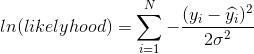，省略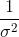可以得到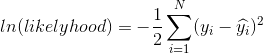。我们只需使得对数似然函数最大即可，因此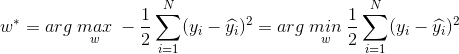。由此我们便退出了线性回归的损失函数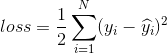，因此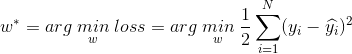，最后只需要对损失函数进行优化即可。在这里我们依旧采用梯度下降法：因为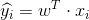，所以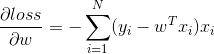，接下来只需按照式子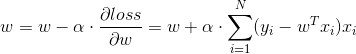更新即可。接下来附上本人使用python编写的linear regression的程序：import numpy as np
from numpy import random as rd
import matplotlib.pyplot as plt
from sklearn.model_selection import train_test_split
from sklearn.metrics import mean_squared_error
rd.seed(2)
np.set_printoptions(linewidth=1000, suppress=True)

def generate_samples(count):
"""

:param count: 样本的个数
:return:
"""
x = rd.uniform(-10, 10, count)
y = 3.5 * x + 4 + rd.uniform(-5, 5, count)
x_return = np.ones((count, 1))
x_return = np.concatenate([x_return, x.reshape(-1, 1)], axis=1)
return x_return, y

class LinearRegression(object):

def __init__(self, count, alpha, lamda, beta, iter_times):
"""

:param lamda: 正则项系数
:param count: 生成样本个数，样本容量
:param alpha: 学习率
:param beta: 学习率衰减系数
:param iter_times: 多少个epoch
"""
self.iter_times = iter_times
self.beta = beta
self.lamda = lamda
self.alpha = alpha
self.x, self.y = generate_samples(count)
self.x_train, self.x_test, self.y_train, self.y_test = train_test_split(self.x, self.y, test_size=0.3, random_state=1)
self.weight = rd.uniform(-10, 10, 2)
self.batch_size = int(0.2 * self.x_train.shape)
self.epoch = int(np.ceil(self.x_train.shape / self.batch_size))
# self.test_mses以及self.train_mses用于存放每一个epoch训练集和测试集的均方误差
self.test_mses = []
self.train_mses = []

def train(self):
for j in range(self.iter_times):
# 第一层循环控制多少个epoch
for i in range(self.epoch):
# 第二层循环完成一次就是一个epoch，每次遍历出一个batch
if i != (self.epoch - 1):
self.x_epoch = self.x_train[i * self.batch_size:(i + 1) * self.batch_size, :]
self.y_epoch = self.y_train[i * self.batch_size:(i + 1) * self.batch_size]
else:
self.x_epoch = self.x_train[i * self.batch_size:, :]
self.y_epoch = self.y_train[i * self.batch_size:]
self.weight -= self.alpha * (-np.sum((self.y_epoch.reshape(-1, 1) - np.dot(self.x_epoch, self.weight.reshape(-1, 1))) * self.x_epoch, axis=0) + 2 * self.lamda * self.weight)
# print(self.weight)
self.alpha *= self.beta
try:
mse_train = mean_squared_error(self.y_train, np.dot(self.x_train, self.weight.reshape(-1, 1)).ravel())
mse_test = mean_squared_error(self.y_test, np.dot(self.x_test, self.weight.reshape(-1, 1)).ravel())
self.train_mses.append(mse_train)
self.test_mses.append(mse_test)
except ValueError as e:
raise Exception("学习率设置过大")
if (j + 1) % int(0.1 * self.iter_times) == 0:
print("================epoch: %d================" % (j + 1))
print("第%d个epoch训练集均方误差：" % (j + 1), mse_train)
print("第%d个epoch测试集均方误差：" % (j + 1), mse_test)

def draw_mses(self):
fig = plt.figure()
ax = plt.subplot(2, 1, 1)
ax2 = plt.subplot(2, 1, 2)
plt.sca(ax)
ax.plot(np.arange(1, 1 + self.iter_times), self.train_mses, label="train dataset mse", color="g")
ax.plot(np.arange(1, 1 + self.iter_times), self.test_mses, label="test dataset mse", color="y")
ax.set_xlabel("epoch")
ax.set_ylabel("mse")
ax.set_title("mse")
ax.legend(loc="upper right")
plt.sca(ax2)
ax2.scatter(self.x[:, 1], self.y, label="samples", marker="*", color="r")
x = np.linspace(-10, 10, 1000).reshape(-1, 1)
x = np.concatenate([np.ones((1000, 1)), x], axis=1)
ax2.plot(x[:, 1].ravel(), np.dot(x, self.weight.reshape(-1, 1)).ravel(), color="b", label="fitness curve")
ax2.set_xlabel("x")
ax2.set_ylabel("y")
ax2.set_title("fitness curve")
ax2.legend(loc="upper left")
plt.show()

def main():
linear = LinearRegression(100, 0.001, 0.8, 0.99, 200)
linear.train()
linear.draw_mses()

if __name__ == "__main__":
main()
展开全文算法
• 单变量线性回归 绘制散点图 相关系数R 拆分训练集和测试集 多变量线性回归 数据检验（判断是否可以做线性回归） 训练线性回归模型 先甩几个典型的线性回归的模型，帮助大家捡起那些年被忘记的数学。 ● 单...
目录

数据导入

单变量线性回归

绘制散点图

相关系数R

拆分训练集和测试集

多变量线性回归

数据检验（判断是否可以做线性回归）

训练线性回归模型

先甩几个典型的线性回归的模型，帮助大家捡起那些年被忘记的数学。

● 单变量线性回归： h(x)=theta0 + theta1* x 1
● 多变量线性回归： h(x)=theta0 + theta1* x 1 + theta2* x 2 + theta3* x 3
● 多项式回归：        h(x)=theta0 + theta1* x 1 + theta2* (x2^2) + theta3* (x3^3)

多项式回归始终还是线性回归，你可以令x2=x2^2，x3=x3^3，简单的数据处理一下就好了，这样上述多项式回归的模型就变成多变量线性回归的模型了。

数据导入

下面我们要开始用数据说话了，先来看看数据源是什么样子吧。

import pandas as pd
import numpy as np
import matplotlib.pyplot as plt
from pandas import DataFrame,Series
from sklearn.cross_validation import train_test_split
from sklearn.linear_model import LinearRegression

#读取文件
datafile = u'E:\\pythondata\\dhdhdh.xlsx'#文件所在位置，u为防止路径中有中文名称，此处没有，可以省略
examDf = DataFrame(data)
examDf.head()

运行结果：

     Call   Connect    Return
0  2.1335  1.267760  1.176615
1  2.5534  1.352941  1.978967
2  3.3361  1.872093  2.249511
3  3.3861  1.826667  2.986597
4  3.9682  1.875000  2.798570

单变量线性回归

绘制散点图

先将上述数据中的Connect（接通量）和Return（回款量）做一个单变量的线性回归，先绘制一个散点图，大致看一下分布情况。

#绘制散点图,examDf.jt为X轴，examDf.hk为Y轴
plt.scatter(examDf.Connect,examDf.Return,color = 'darkgreen',label = "Exam Data")

#添加图的标签（x轴，y轴）
plt.xlabel("The Connection amount of the average account")#设置X轴标签
plt.ylabel("The ratio of average return amount")#设置Y轴标签
plt.show()#显示图像

运行结果：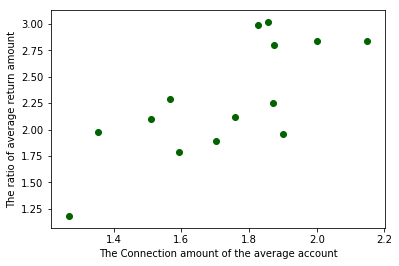看来商业中的实际数据总是和课本上的完美数据不一样，看这零零散散的分布，线性关系很是勉强，但是大致还是有一个线性相关的样子的，那么就看看相关系数有多少吧。

相关系数R

rDf = examDf.corr()#查看数据间的相关系数
print(rDf)

运行结果：

             Call   Connect    Return
Call     1.000000  0.445870  0.441673
Connect  0.445870  1.000000  0.745338
Return   0.441673  0.745338  1.000000

Connect（接通量）和Return（回款量）的相关系数为0.745338，还不错。

相关系数是用以反映变量之间相关关系密切程度的统计指标，对于相关性强度来说，我们一般认为：

0~0.3 弱相关

0.3~0.6  中等程度相关

0.6~1  强相关

在数学中，相关系数的计算一般是这样的，给数学狂人看：

R(相关系数) = X和Y的协方差 / (X的标准差 * Y的标准差) == cov（X,Y）/ σX * σY （即person系数）

拆分训练集和测试集

Connect（接通量）和Return（回款量）属于强相关，可以进行线性回归训练，那么我们先来拆分训练集和测试集吧。

#拆分训练集和测试集（train_test_split是存在与sklearn中的函数）
X_train,X_test,Y_train,Y_test = train_test_split(examDf.Connect,examDf.Return,train_size=0.8)
#train为训练数据,test为测试数据,examDf为源数据,train_size 规定了训练数据的占比

print("自变量---源数据:",examDf.Connect.shape, "；  训练集:",X_train.shape, "；  测试集:",X_test.shape)
print("因变量---源数据:",examDf.Return.shape, "；  训练集:",Y_train.shape, "；  测试集:",Y_test.shape)

#散点图
plt.scatter(X_train, Y_train, color="darkgreen", label="train data")#训练集为深绿色点
plt.scatter(X_test, Y_test, color="red", label="test data")#测试集为红色点

#添加标签
plt.legend(loc=2)#图标位于左上角，即第2象限，类似的，1为右上角，3为左下角，4为右下角
plt.xlabel("The Connection amount of the average account")#添加 X 轴名称
plt.ylabel("The ratio of average return amount")#添加 Y 轴名称
plt.show()#显示散点图

运行结果：

自变量---源数据: (14,) ；  训练集: (11,) ；  测试集: (3,)
因变量---源数据: (14,) ；  训练集: (11,) ；  测试集: (3,)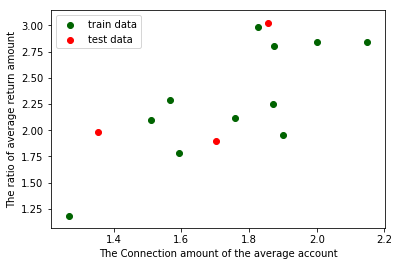回归模型训练

#调用线性规划包
model = LinearRegression()

#线性回归训练
model.fit(X_train,Y_train)#调用线性回归包

a  = model.intercept_#截距
b = model.coef_#回归系数

#训练数据的预测值
y_train_pred = model.predict(X_train)
#绘制最佳拟合线：标签用的是训练数据的预测值y_train_pred
plt.plot(X_train, y_train_pred, color='blue', linewidth=2, label="best line")

#测试数据散点图
plt.scatter(X_train, Y_train, color='darkgreen', label="train data")
plt.scatter(X_test, Y_test, color='red', label="test data")

#添加图标标签
plt.legend(loc=2)#图标位于左上角，即第2象限，类似的，1为右上角，3为左下角，4为右下角
plt.xlabel("The Connection amount of the average account")#添加 X 轴名称
plt.ylabel("The ratio of average return amount")#添加 Y 轴名称
plt.show()#显示图像

print("拟合参数:截距",a,",回归系数：",b)
print("最佳拟合线: Y = ",round(a,2),"+",round(b,2),"* X")#显示线性方程，并限制参数的小数位为两位


运行结果：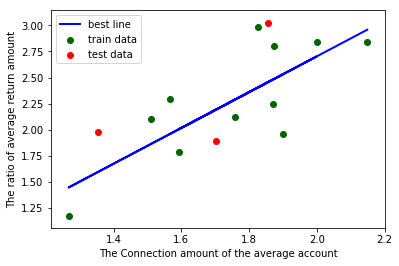拟合参数:截距 -0.7291766860887745 ,回归系数： [1.71584366]
最佳拟合线: Y =  -0.73 + 1.72 * X

补充一句，有博友说单变量线性回归上面的这段代码中，加一个reshape就能运行出来了，可能是版本不同，我没加也能运行出来，所以分享在下面给大家参考一下，如果有和博友一样的情况，可以试试增加下面一段代码（谢谢博友 dsy23 的分享）：


#调用线性规划包
model = LinearRegression()

#在这里加一段
X_train = X_train.values.reshape(-1,1)
X_test = X_test.values.reshape(-1,1)

#线性回归训练
model.fit(X_train,Y_train)#调用线性回归包

a  = model.intercept_#截距
b = model.coef_#回归系数

多变量线性回归

在单变量线性回归中，我们将每一步都讲解的极其详细，所以在多变量线性回归中，我们不会重复讲那些简单的部分了，但是为了防止python小白迷失自己，所以在这部分该有的代码还是会甩出来，该有的备注也都有，只不过不会一点一点分步骤来了。

上面我们提到多变量线性回归的模型为h(x)=theta0 + theta1* x 1 + theta2* x 2 + theta3* x 3，下面，我们还是使用单变量线性回归中的数据，单变量线性回归中，我们只用到了Connect（接通量）和Return（回款量），既然是多变量回归模型，那么我们就多加一个变量Call（拨打量）。

数据检验（判断是否可以做线性回归）

#-*- coding:utf-8 -*-

import pandas as pd
import numpy as np
import seaborn as sns
import matplotlib.pyplot as plt
from pandas import DataFrame,Series
from sklearn.cross_validation import train_test_split
from sklearn.linear_model import LinearRegression

#读取文件
datafile = u'E:\\pythondata\\dhdhdh.xlsx'#文件所在位置，u为防止路径中有中文名称，此处没有，可以省略
examDf = DataFrame(data)

#数据清洗,比如第一列有可能是日期，这样的话我们就只需要从第二列开始的数据，
#这个情况下，把下面中括号中的0改为1就好，要哪些列取哪些列
new_examDf = examDf.ix[:,0:]

#检验数据
print(new_examDf.describe())#数据描述，会显示最值，平均数等信息，可以简单判断数据中是否有异常值
print(new_examDf[new_examDf.isnull()==True].count())#检验缺失值，若输出为0，说明该列没有缺失值

#输出相关系数，判断是否值得做线性回归模型
print(new_examDf.corr())#0-0.3弱相关；0.3-0.6中相关；0.6-1强相关；

#通过seaborn添加一条最佳拟合直线和95%的置信带，直观判断相关关系
sns.pairplot(data, x_vars=['Call','Connect'], y_vars='Return', size=7, aspect=0.8, kind='reg')
plt.show()


运行结果：

            Call    Connect     Return
count  99.000000  99.000000  99.000000
mean    3.153649   1.967779   2.591121
std     1.027607   0.470364   0.790172
min     1.280100   1.014208   0.941292
25%     2.373528   1.596610   2.044147
50%     3.040000   1.913472   2.476091
75%     3.835980   2.253994   3.035603
max     5.851181   3.234977   5.160840
Call       0
Connect    0
Return     0
dtype: int64
Call   Connect    Return
Call     1.000000  0.837052  0.744792
Connect  0.837052  1.000000  0.844640
Return   0.744792  0.844640  1.000000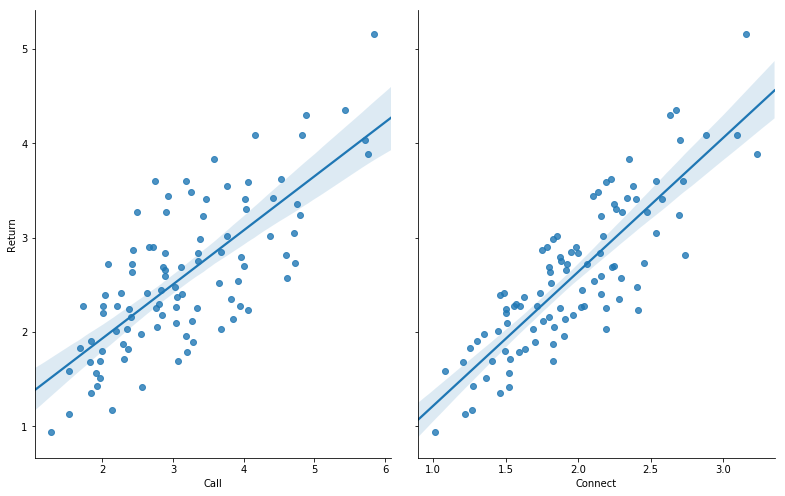判断是否可以做线性回归：

异常值：通过最值或者平均数或者中位数等判断，或者直接通过查看是否有游离在大部队之外的点来判断是否有异常值；
空值：这个没办法，你必须看运行结果的10-12行是否等于0，是0则无空值，如果不是0，就要看看是删掉空值呢？还是用其他值代替呢？不同情况不同对待；
相关性：要么通过相关系数的大小判断，要么看散点图中的最佳拟合直线和95%的置信带，直观判断相关关系；

训练线性回归模型

#拆分训练集和测试集
X_train,X_test,Y_train,Y_test = train_test_split(new_examDf.ix[:,:2],new_examDf.Return,train_size=0.8)
#new_examDf.ix[:,:2]取了数据中的前两列为自变量，此处与单变量的不同

print("自变量---源数据:",new_examDf.ix[:,:2].shape, "；  训练集:",X_train.shape, "；  测试集:",X_test.shape)
print("因变量---源数据:",examDf.Return.shape, "；  训练集:",Y_train.shape, "；  测试集:",Y_test.shape)

#调用线性规划包
model = LinearRegression()

model.fit(X_train,Y_train)#线性回归训练

a  = model.intercept_#截距
b = model.coef_#回归系数
print("拟合参数:截距",a,",回归系数：",b)

#显示线性方程，并限制参数的小数位为两位
print("最佳拟合线: Y = ",round(a,2),"+",round(b,2),"* X1 + ",round(b,2),"* X2")

Y_pred = model.predict(X_test)#对测试集数据，用predict函数预测

plt.plot(range(len(Y_pred)),Y_pred,'red', linewidth=2.5,label="predict data")
plt.plot(range(len(Y_test)),Y_test,'green',label="test data")
plt.legend(loc=2)
plt.show()#显示预测值与测试值曲线


运行结果：

自变量---源数据: (99, 2) ；  训练集: (79, 2) ；  测试集: (20, 2)
因变量---源数据: (99,) ；  训练集: (79,) ；  测试集: (20,)
拟合参数:截距 -0.006540954521370601 ,回归系数： [0.08624344 1.19029593]
最佳拟合线: Y =  -0.01 + 0.09 * X1 +  1.19 * X2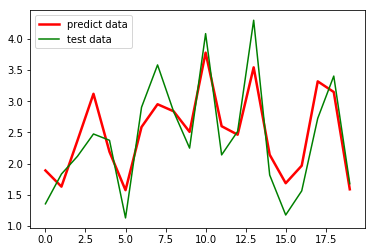效果勉强满意，毕竟才用了80个数训练的模型，要求高的话多用一些数据就好。

除了用 Python 做线性回归，我们还可以使用 SPSS 来做，不用编程，不用各种调参，就是点几下就可以输出结果，更简便！具体操作请参考下面链接里的文章：

数据建模 - SPSS做多元线性回归 - 分析步骤、输出结果详解、与Python的结果对比 -（SPSS）

【此处是重点】打赏一下发数据！


展开全文python 回归分析
• 最近做了一些用SPSS进行线性回归的实验，还是感觉很多细节把握不好，这里结合我的实验结果，以及网上别人的介绍总结一下，先贴几张SPSS的输出： 下面简单解释一下这三张图中的结果： 第一个表模型汇总表中，...
先说一句题外话，如果当年在大学里数理统计等课程结合SPSS，SAS，R等软件来讲，应该效果会好很多。

最近做了一些用SPSS进行线性回归的实验，还是感觉很多细节把握不好，这里结合我的实验结果，以及网上别人的介绍总结一下，先贴几张SPSS的输出：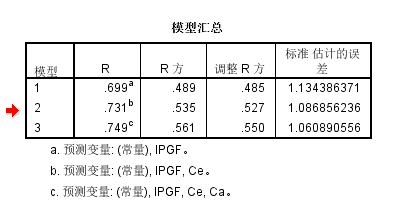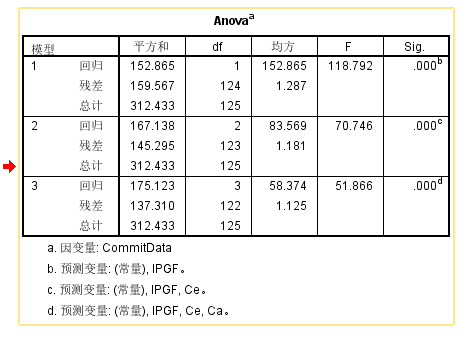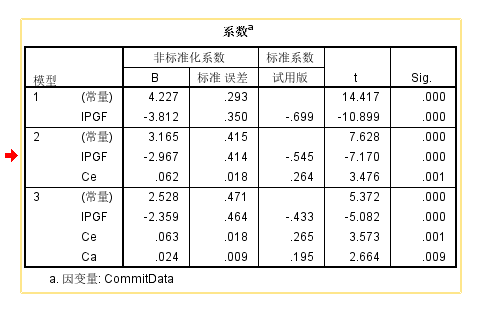下面简单解释一下这三张图中的结果：

第一个表模型汇总表中，R表示拟合优度（goodness of fit），它是用来衡量估计的模型对观测值的拟合程度。它的值越接近1说明模型越好。调整的R平方比调整前R平方更准确一些，图中的最终调整R方为0.550，表示自变量一共可以解释因变量55%的变化（variance），另外，由于使用的是StepWise Linear Regression (SWLR)，分析——回归——线性——“方法”选择“逐步”，所以模型1、2、3的R方逐渐增大，标准误差逐渐减小。

（据网友的介绍：一般认为，拟合优度达到0.1为小效应（R方0.01），0.3为中等（R方0.09），0.5为大（R方0.25），这是针对自然科学的一般界限。）

第二个表Anova表示方差分析结果，主要看F和sig值两个，F值为方差分析的结果，是一个对整个回归方程的总体检验，指的是整个回归方程有没有使用价值（与随机瞎猜相比），其F值对应的Sig值小于0.05就可以认为回归方程是有用的。另外，从F值的角度来讲：F的值是回归方程的显著性检验，表示的是模型中被解释变量与所有解释变量之间的线性关系在总体上是否显著做出推断。若F>Fa(k,n-k-1)，则拒绝原假设，即认为列入模型的各个解释变量联合起来对被解释变量有显著影响，反之，则无显著影响。

这里简单对Fa(k,n-k-1)进行一下解释，k为自变量个数，n为样本容量，n-k-1为自由度。对于我的实验中的情况来讲，k=3，样本容量为146，所以查表的时候应该差Fa(3,142)，一般数理统计课本中都有F分布表，a表示的显著性水平（一般取0.05），但我们手头不一定会有课本，就需要借助于excel来查F表，打开excel，在公式区输入：=FINV(0.05,3,142)，在单元格中即出现2.668336761，表中的F值显著大于这个值，则认为各个解释变量对因变量有显著影响。

需要注意的是，方差分析是对多个自变量的总体检验，而不是单个自变量（单个自变量在系数表中，为单样本T检验），这就是第三个表回归系数表中的内容。

系数表格列出了自变量的显著性检验结果（使用单样本T检验），最后一列为T检验的sig，表中均小于0.05，说明自变量对因变量具有显著影响，B表示各个自变量在回归方程中的系数，负值表示IPGF这个自变量对因变量有显著的负向影响，但是由于每个自变量的量纲和取值范围不同，基于B并不能反映各个自变量对因变量影响程度的大小，这时候我们就要借助标准系数。目前表格中的“试用版”实际上是Beta的意思，此时数值越大表示对自变量的影响更大。

从这个分析过程来看，这个实验结果还挺理想的。

展开全文• 当只有一个自变量时，称为简单线性回归，当具有多个自变量时，称为多元线性回归。 线性关系的理解： 画出来的图像是直的。 每个自变量的最高次项为1。 拟合是指构建一种算法，使得该算法能够符合真实的数据。从...
回归分析是用来评估变量之间关系的统计过程。用来解释自变量X与因变量Y的关系。即当自变量X发生改变时，因变量Y会如何发生改变。
线性回归是回归分析的一种，评估的自变量X与因变量Y之间是一种线性关系。当只有一个自变量时，称为简单线性回归，当具有多个自变量时，称为多元线性回归。
线性关系的理解：

画出来的图像是直的。
每个自变量的最高次项为1。

拟合是指构建一种算法，使得该算法能够符合真实的数据。从机器学习角度讲，线性回归就是要构建一个线性函数，使得该函数与目标值之间的相符性最好。从空间的角度来看，就是要让函数的直线（面），尽可能靠近空间中所有的数据点（点到直线的平行于y轴的距离之和最短）。线性回归会输出一个连续值。
线性回归模型
简单线性回归
我们可以以房屋面积（x）与房价（y）为例，二者是线性关系，房屋价格正比于房屋面积，假设比例为w：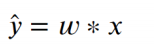然而，这种线性方程一定是过原点的，即x为0时，y也一定为0。这可能并不符合现实中某些场景。为了能够让方程具有更广泛的适应性，就要再增加一个截距，设为b，则方程可以变为：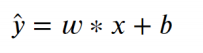以上方程就是数据建模的模型，w与b就是模型的参数。
线性回归是用来解释自变量与因变量之间的关系，但这种关系并非严格的函数映射关系。
多元线性回归
现实中的数据可能是比较复杂的，自变量也可能不止一个，例如，影响房屋价格也很可能不止房屋面积一个因素，可能还有是否在地铁附近，房间数，层数，建筑年代等诸多因素。不过，这些因素对房价影响的权重是不同的，因此，我们可以使用多个权重来表示多个因素与房屋价格的关系：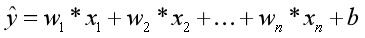x：影响因素，即特征。
w：每个x的影响力度。
n：特征的个数。
y^：房屋的预测价格。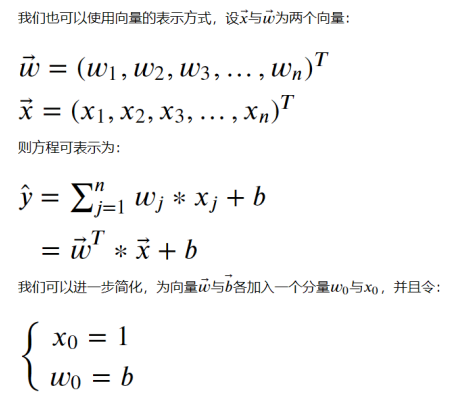这样，就可以表示为：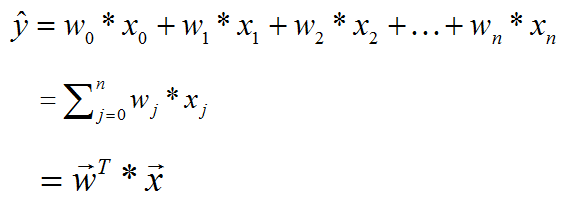多元线性回归在空间中，可以表示为一个超平面，去拟合空间中的数据点。
我们的目的就是从现有的数据中，去学习w与b的值。一旦w与b的值确定，就能够确定拟合数据的线性方程，这样就可以对未知的数据x进行预测y（房价）。
损失函数与参数求解
损失函数
对于机器学习来讲，就是从已知数据去建立一个模型，使得该模型能够对未知数据进行预测。实际上，机器学习的过程就是确定模型参数的过程。
对于监督学习来说，我们可以通过建立损失函数来实现模型参数的求解，损失函数也称目标函数或代价函数，简单来说就是关于误差的一个函数。损失函数用来衡量模型预测值与真实值之间的差异。机器学习的目标，就是要建立一个损失函数，使得该函数的值最小。
也就是说，损失函数是一个关于模型参数的函数（以模型参数w作为自变量的函数），自变量可能的取值组合通常是无限的，我们的目标，就是要在众多可能的组合中，找到一组最合适的自变量组合，使得损失函数的值最小。
在线性回归中，我们使用平方损失函数（最小二乘法），定义如下：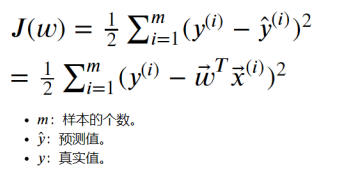简单线性回归程序
我们以鸢尾花数据集中花瓣长度与花瓣宽度为例，通过程序实现简单线性回归。
import numpy as np
#用于线性回归的类
from sklearn.linear_model import LinearRegression
#用来切分训练集与测试集
from sklearn.model_selection import train_test_split
#设置输出精度。默认为8
np.set_printoptions(precision=2)

#获取花瓣长度作为x，花瓣宽度作为y。
x, y = iris.data[:,2].reshape(-1,1), iris.data[:,3]

lr = LinearRegression()
x_train, x_test, y_train, y_test = train_test_split(x, y, test_size=0.25, random_state=0)
#使用训练集数据，训练模型。
lr.fit(x_train, y_train)
print('权重：', lr.coef_)
print('截距：', lr.intercept_)
y_hat = lr.predict(x_test)
print('实际值：', y_test[:5])
print('预测值：', y_test[:5])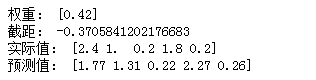import matplotlib.pyplot as plt

plt.rcParams['font.family'] = 'SimHei'
plt.rcParams['axes.unicode_minus'] = False
plt.rcParams['font.size'] = 15

plt.figure(figsize=(10,6))
plt.scatter(x_train, y_train, c='orange', label='训练集')
plt.scatter(x_test, y_test, c='g', marker='D', label='测试集')
plt.plot(x, lr.predict(x), 'r-')
plt.legend()
plt.xlabel('花瓣长度')
plt.ylabel('花瓣宽度')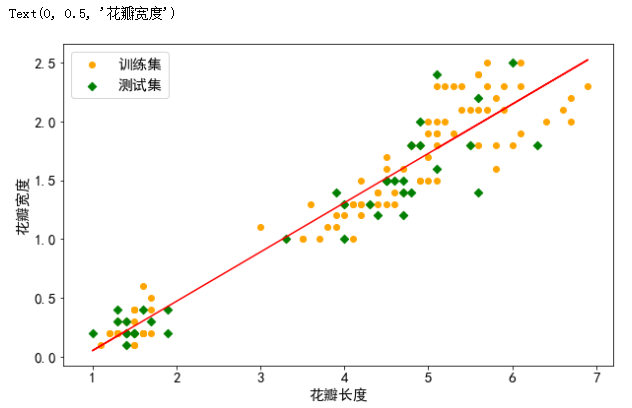plt.figure(figsize=(15,6))
plt.plot(y_test, label='真实值', color='r', marker='o')
plt.plot(y_hat, c='g', marker='o', ls='--', label='预测值')
plt.legend()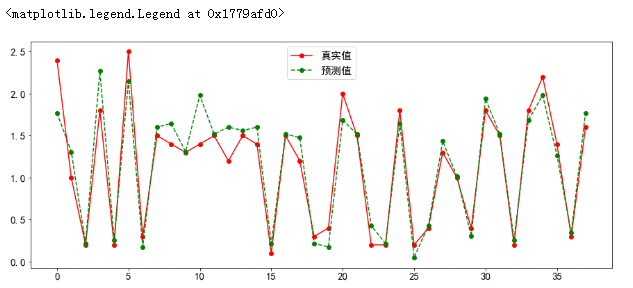回归模型预测
对于回归模型，我们可以采用如下指标进行衡量。

MSE
RMSE
MAE
R2

MSE
MSE（Mean Squared Error），平均平方差，为所有样本数据误差（真实值与预测值之差）的平方和，然后取均值。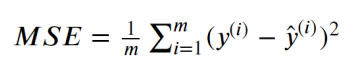RMSE
RMSE（Root Mean Squared Error），平均平方误差的平方根，即在MSE的基础上，取平方根。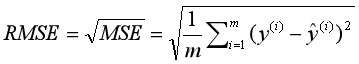MAE
MAE（Mean Absolute Error），平均绝对值误差，为所有样本数据误差的绝对值和。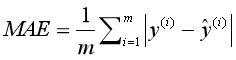R2
R2为决定系数，用来表示模型拟合性的分值，值越高表示模型拟合性越好，在训练集中，R2的取值范围为[0,1]。在R2的取值范围为[-∞,1]。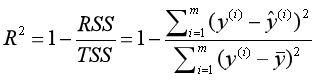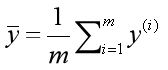from sklearn.metrics import mean_squared_error, mean_absolute_error, r2_score

print('均方误差（MSE）：',mean_squared_error(y_test,y_hat))
print('根均方误差（RMSE）：',np.sqrt(mean_squared_error(y_test,y_hat)))
print('平均绝对值误差（MAE）：',mean_absolute_error(y_test,y_hat))
print('训练集R^2：',r2_score(y_train,lr.predict(X_train)))
print('测试集R^2：',r2_score(y_test,y_hat))
#lr.score其实求解的就是R^2的值，但需要注意，r2_score方法与lr.score方法传递参数的内容是不同的。
print('训练集R^2：',lr.score(X_train,y_train))
print('测试集R^2：',lr.score(X_test,y_test))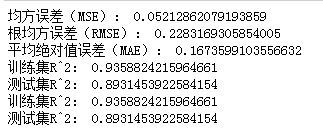多元线性回归程序
我们可以以波士顿房价为例来做程序演示
from sklearn.linear_model import LinearRegression
from sklearn.model_selection import train_test_split
import pandas as pd

x, y = boston.data, boston.target
df = pd.DataFrame(np.concatenate([x, y.reshape(-1,1)], axis=1),
columns = boston.feature_names.tolist()+['MEDV'])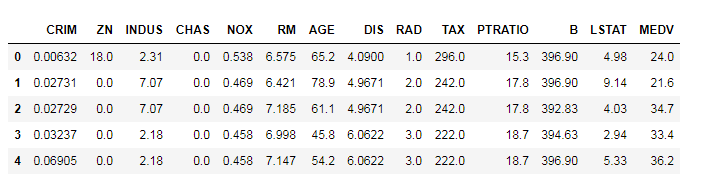x_train, x_test, y_train, y_test = train_test_split(x, y, test_size=0.25, random_state=0)
lr = LinearRegression()
lr.fit(x_train, y_train)
y_hat = lr.predict(x_test)
print('权重：',lr.coef_)
print('截距：',lr.intercept_)
print('实际值：',y_test[:10])
print('测试值：',y_hat[:10])
print('训练值R^2:',lr.score(X_train, y_train))
print('测试值R^2:',lr.score(X_test, y_test))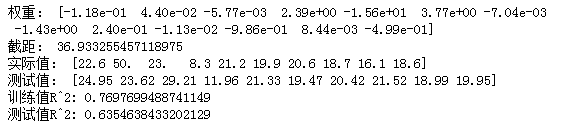展开全文机器学习 python 算法
• 本课程主要讲述如何使用python进行线性回归与非线性回归分析，包括： 基于statsmodel的线性回归方法 基于sklearn的线性回归方法 基于Numpy的一元多项式非线性回归方法 ...大数据 大数据技术 大数据平台 大数据技术原理与应用 大数据学习路线
• 本文致力于手把手教你实现一个最简单的机器学习模型--一元线性回归模型。短短的14行代码，就实现了。希望读完以后，你也能自己实现它。并对线性回归有更好的了解，或者从不了解到了解。Python 算法
• 本文是从一元线性回归为为基础来理解线性回归，适合于线性回归的入门，让初学者对于线性回归有直观的理解。本文也是我对于线性回归算法入门学习，分享给大家。 线性回归的定义 回归是用于应用于输入变量与输出...机器学习 梯度下降
• 机器学习(三)线性回归模型、广义线性回归模型、非线性回归模型   线性回归（数据集要满足正态分布） 一元线性回归模型： 在这里会想到，如何确定方程中的系数呢？我们先来了解最小二乘法，简单来说就是这个点...机器学习
• 一元线性回归和多元线性回归表面意思容易理解，但是结合实际的数据集，会混乱。这也是在编写线性回归博文的时候梳理知识点发现自己的不足，同时记录下来，让有疑问的同学也可以得到答案，拨开乌云。 1.在数据集上的...
• 线性回归action精讲 线性回归是利用数理统计中回归分析，来确定两种或两种以上变量间相互依赖的定量关系的一种统计分析方法，运用十分广泛。其表达形式为y = w’x+e，e为误差服从均值为0的正态分布。 一元与多元 ...
• 线性回归线性回归的延伸，线性就是每个变量的指数都是 1，而非线性就是至少有一个变量的指数不是 1。生活中，很多现象之间的关系往往不是线性关系。选择合适的曲线类型不是一件轻而易举的工作，主要依靠专业知识...
• 回归分析属于有监督学习问题，本博客将重点回顾标准线性回归知识点，并就线性回归中可能出现的问题进行简单探讨，引出线性回归的两个变种岭回归以及Lasso回归，最后通过sklearn库模拟整个回归过程。 目录结构 线性...机器学习 Lasso回归
• 在上一篇文章中我们介绍了 回归分析之理论篇，在其中我们有聊到线性回归和非线性回归，包括广义线性回归，这一篇文章我们来聊下回归分析中的线性回归回归分析 机器学习 python...